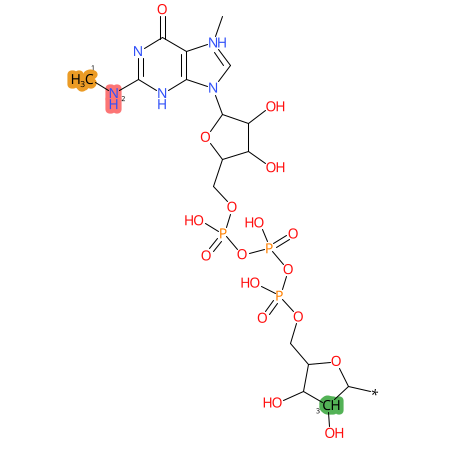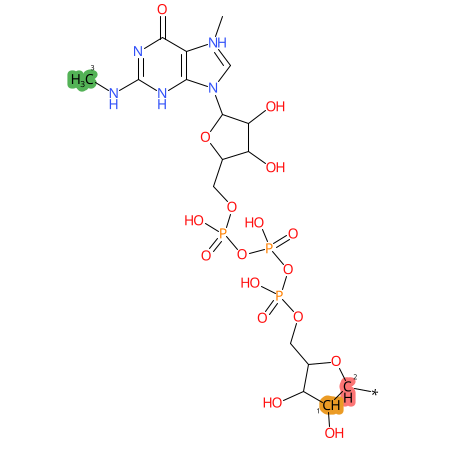Modomics - A Database of RNA Modifications

### Summary

 Full name N2,7-dimethylguanosine cap (cap DMG) Short name m2,7GpppN MODOMICS code 279553N Nature of the modified residue Natural RNAMods code ® Residue unique ID 133 Found in RNA Yes Enzymes Tgs1 (Homo sapiens) Found in phylogeny Eukaryota

### Chemical information

 Sum formula C17H25N5O17P3 Type of moiety nucleotide Degeneracy unspecified residue SMILES CNc1nc(=O)c2c([n](c[n+]2C)[C@@H]2O[C@H](COP([O-])(OP([O-])(OP([O-])(OC[C@H]3O[C@@H]%91[C@H](O)[C@@H]3O)=O)=O)=O)[C@@H](O)[C@H]2O)[nH]1.[*]%91 logP -1.6189 TPSA 352.71 Number of atoms 43 Number of Hydrogen Bond Acceptors 1 (HBA1) 17 Number of Hydrogen Bond Acceptors 2 (HBA2) 20 Number of Hydrogen Bond Donors (HBD) 6 PDB no exac match , link to the most similar ligand GTG HMDB (Human Metabolome Database) no exac match, link to the most similar ligand HMDB0001273 InChI InChIKey

* Chemical properties calculated with Open Babel - O'Boyle et al. Open Babel: An open chemical toolbox. J Cheminform 3, 33 (2011) (link)

 2D .png .mol .mol2 .sdf .pdb .smi 3D .mol .mol2 .sdf .pdb

### Tautomers

 Tautomers SMILES CN=c1[nH]c(=O)c2c(n(c[n+]2C)C3OC(COP([O-])(OP([O-])(OP([O-])(OCC4OC(C(O)C4O)*)=O)=O)=O)C(O)C3O)[nH]1 tautomer #0 CNc1[nH]c(=O)c2c(n(c[n+]2C)C3OC(COP([O-])(OP([O-])(OP([O-])(OCC4OC(C(O)C4O)*)=O)=O)=O)C(O)C3O)n1 tautomer #1 CNc1nc(O)c2c(n(c[n+]2C)C3OC(COP([O-])(OP([O-])(OP([O-])(OCC4OC(C(O)C4O)*)=O)=O)=O)C(O)C3O)n1 tautomer #2 CNc1nc(=O)c2c(n(c[n+]2C)C3OC(COP([O-])(OP([O-])(OP([O-])(OCC4OC(C(O)C4O)*)=O)=O)=O)C(O)C3O)[nH]1 tautomer #3 CN=c1[nH]c(O)c2c(n(c[n+]2C)C3OC(COP([O-])(OP([O-])(OP([O-])(OCC4OC(C(O)C4O)*)=O)=O)=O)C(O)C3O)n1 tautomer #4 CNc1nc(O)c2c(n(c[n+]2C)C3OC(COP([O-])(OP([O-])(OP([O-])(OCC4OC(C(O)C4O)*)=O)=O)=O)C(O)C3O)n1 tautomer #5 CN=C1NC(=O)C2C(N(C=[N+]2C)C3OC(COP([O-])(OP([O-])(OP([O-])(OCC4OC(C(O)C4O)*)=O)=O)=O)C(O)C3O)=N1 tautomer #6 CN=c1nc(O)c2c(n(c[n+]2C)C3OC(COP([O-])(OP([O-])(OP([O-])(OCC4OC(C(O)C4O)*)=O)=O)=O)C(O)C3O)[nH]1 tautomer #7 CNC1=NC(=O)C2C(N(C=[N+]2C)C3OC(COP([O-])(OP([O-])(OP([O-])(OCC4OC(C(O)C4O)*)=O)=O)=O)C(O)C3O)=N1 tautomer #8 CN=C1N=C(O)C2C(N(C=[N+]2C)C3OC(COP([O-])(OP([O-])(OP([O-])(OCC4OC(C(O)C4O)*)=O)=O)=O)C(O)C3O)=N1 tautomer #9 Tautomer image Show Image

### Predicted CYP Metabolic Sites

CYP3A4 CYP2D6 CYP2C9* CYP Metabolic sites predicted with SMARTCyp. SMARTCyp is a method for prediction of which sites in a molecule that are most liable to metabolism by Cytochrome P450. It has been shown to be applicable to metabolism by the isoforms 1A2, 2A6, 2B6, 2C8, 2C19, 2E1, and 3A4 (CYP3A4), and specific models for the isoform 2C9 (CYP2C9) and isoform 2D6 (CYP2D6). CYP3A4, CYP2D6, and CYP2C9 are the three of the most important enzymes in drug metabolism since they are involved in the metabolism of more than half of the drugs used today. The three top-ranked atoms are highlighted. See: SmartCYP and SmartCYP - background; Patrik Rydberg, David E. Gloriam, Lars Olsen, The SMARTCyp cytochrome P450 metabolism prediction server, Bioinformatics, Volume 26, Issue 23, 1 December 2010, Pages 2988–2989 (link)

### LC-MS Information

 Monoisotopic mass 469.04 Average mass 664.325 [M+H]+ not available Product ions not available Normalized LC elution time * not available LC elution order/characteristics not available

* normalized to guanosine (G), measured with a RP C-18 column with acetonitrile/ammonium acetate as mobile phase.

As with the m7G cap, the m2,7G cap is indistinguishable from any other m7m2G apart from the triester linkage linking it to the rest of the RNA chain, and I'm putting it here separately just for orderliness purpose.

### Chemical groups contained

TypeSubtype
methyl groupmethyl at aromatic N

### Reactions starting from N2,7-dimethylguanosine cap (cap DMG)

Last modification of this entry: Sept. 29, 2021